06-05-2015

## Linear models

Explain a variable y as a linear function of x (and z, etc.).

`model <- lm(dist ~ speed, data=cars)`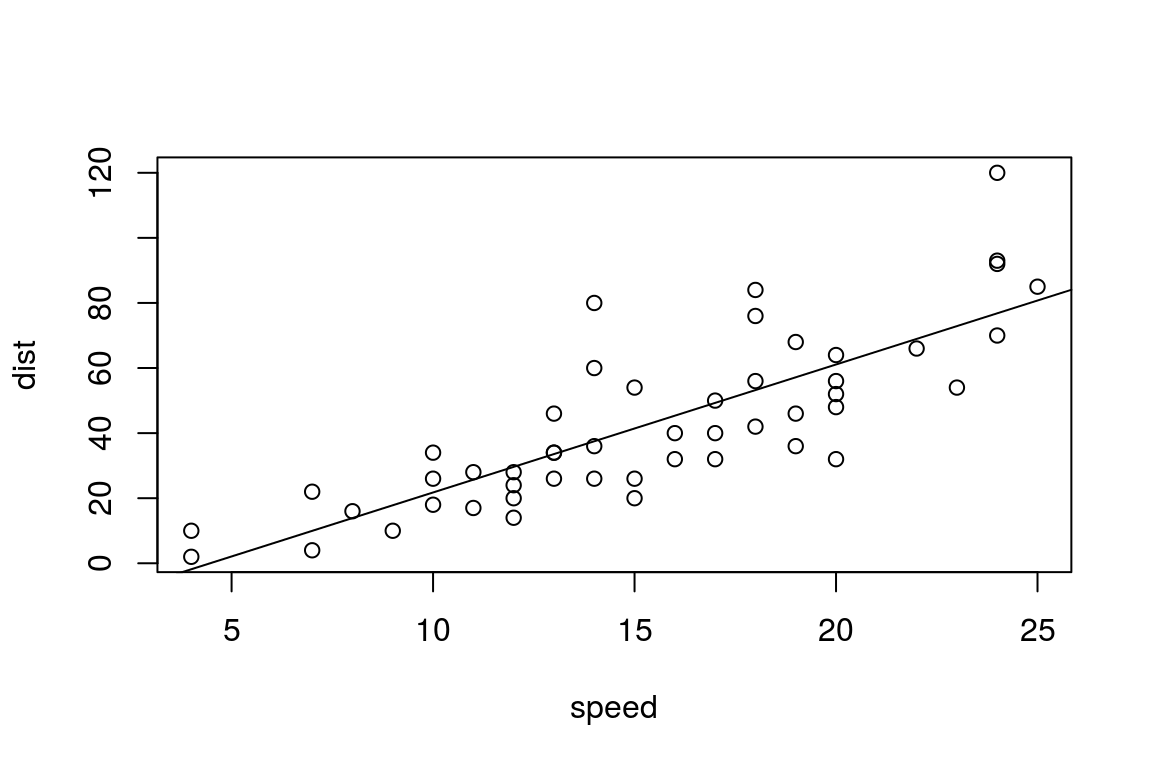## Assumptions

• Normal of errors
• Independence of observations

## Residual of errors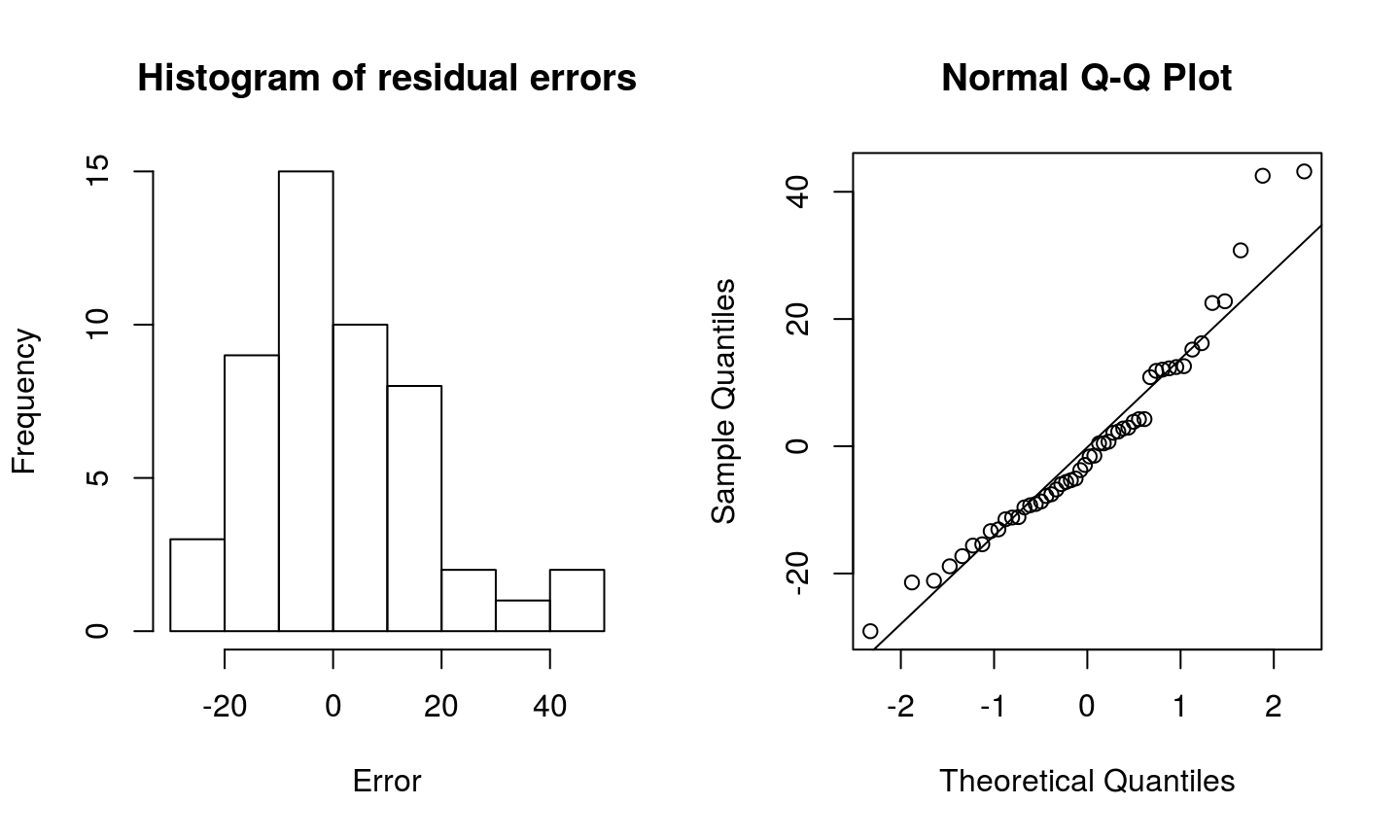## Independence of observations

`head(my.cars)   #See first observations`
```##   speed dist id
## 1     4    2  1
## 2     4   10  2
## 3     7    4  3
## 4     7   22  4
## 5     8   16  5
## 6     9   10  6```
`str(my.cars)    #See datatypes in dataframe`
```## 'data.frame':    50 obs. of  3 variables:
##  \$ speed: num  4 4 7 7 8 9 10 10 10 11 ...
##  \$ dist : num  2 10 4 22 16 10 18 26 34 17 ...
##  \$ id   : Factor w/ 50 levels "1","2","3","4",..: 1 2 3 4 5 6 7 8 9 10 ...```

## Pseudoreplications

`head(my.cars)   #See first observations`
```##   speed dist id
## 1     4    2  1
## 2     4   10  1
## 3     7    4  1
## 4     7   22  1
## 5     8   16  1
## 6     9   10  1```
`str(my.cars)    #See datatypes in dataframe`
```## 'data.frame':    50 obs. of  3 variables:
##  \$ speed: num  4 4 7 7 8 9 10 10 10 11 ...
##  \$ dist : num  2 10 4 22 16 10 18 26 34 17 ...
##  \$ id   : Factor w/ 5 levels "1","2","3","4",..: 1 1 1 1 1 1 1 1 1 1 ...```

## Pseudoreplications

• Several observations from one participant:
• Repeated measure design
• Time courses across participants
• Group/sample cluster

## Solutions

• Summary statistics
• Mixed models

## Summary or multilevel

A dataset: How boring is it to participate in a psychophysics experiment?

`summary(data)   #summary of data`
```##      rating            id
##  Min.   :1.000   1      :10
##  1st Qu.:3.933   2      :10
##  Median :5.065   3      :10
##  Mean   :5.133   4      :10
##  3rd Qu.:6.624   5      :10
##  Max.   :9.843   6      :10
##                  (Other):40```

## Summary or multilevel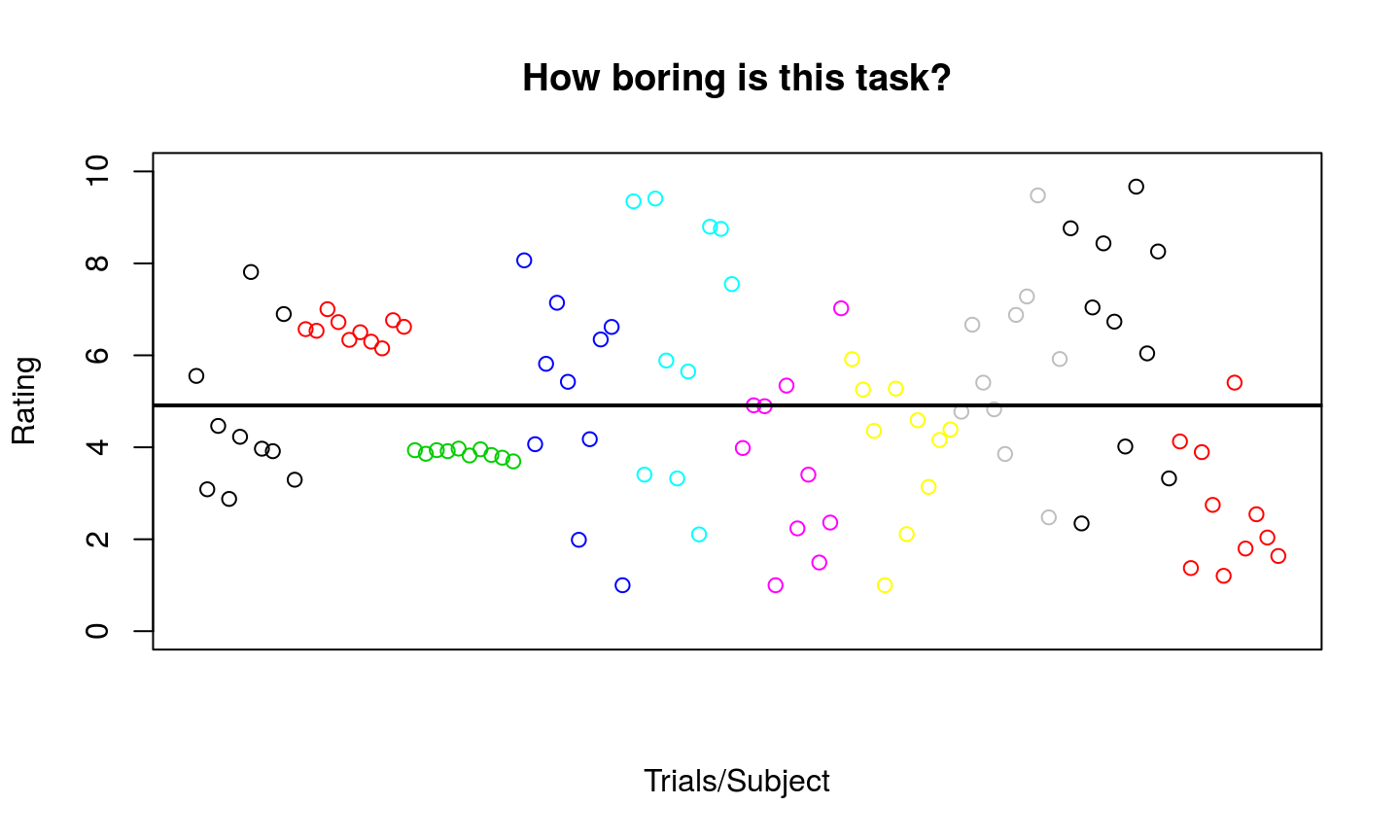## Summary or multilevel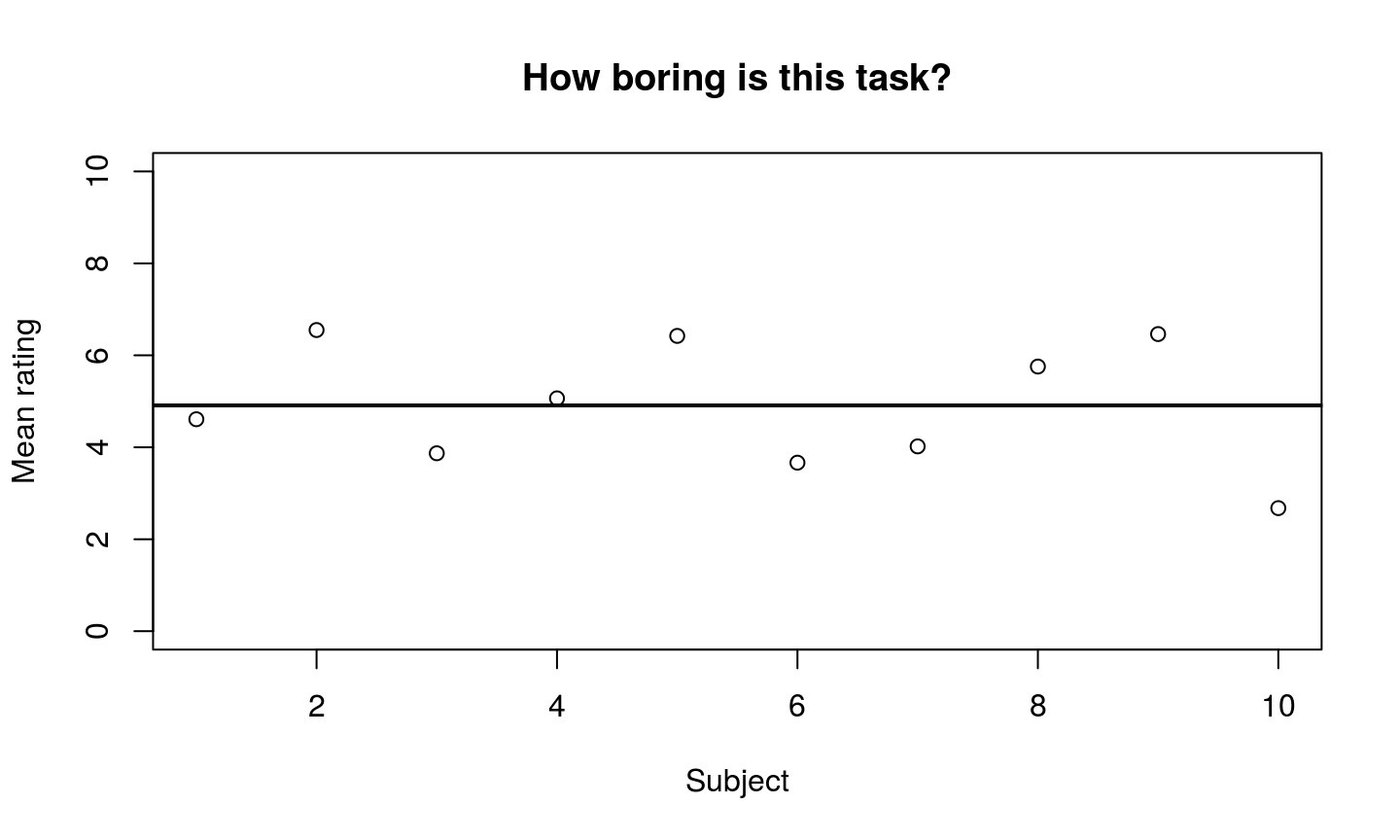## Summary or multilevel

Calculate mean of rating per subject, then model.

```summary.data1 <- tapply(rating2,id,mean)
summary.model1 <- lm(summary.data~1)     ```

Make a linear mixed model using subject as random effect

```library(lme4)  #First load package "lme4"
mixed.model1 <- lmer(rating2~1+(1|id))   ```

## Summary or multilevel

`display(summary.model1)`
```## lm(formula = summary.data ~ 1)
##             coef.est coef.se
## (Intercept) 4.91     0.43
## ---
## n = 10, k = 1
## residual sd = 1.36, R-Squared = 0.00```

## Summary or multilevel

`display(mixed.model1)`
```## lmer(formula = rating2 ~ 1 + (1 | id))
## coef.est  coef.se
##     4.91     0.43
##
## Error terms:
##  Groups   Name        Std.Dev.
##  id       (Intercept) 1.23
##  Residual             1.83
## ---
## number of obs: 100, groups: id, 10
## AIC = 426.4, DIC = 420.6
## deviance = 420.5```

## Summary or multilevel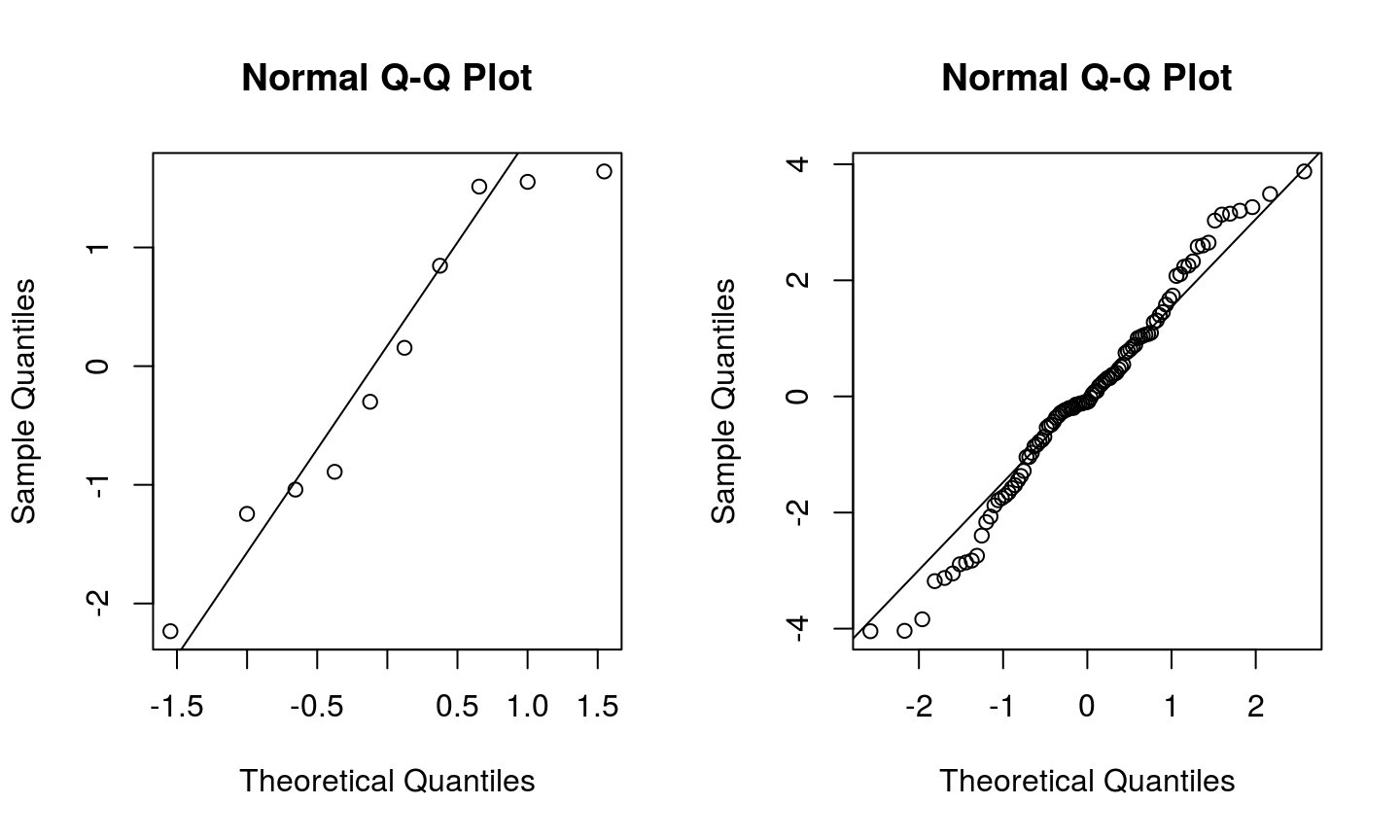## Unbalanced designs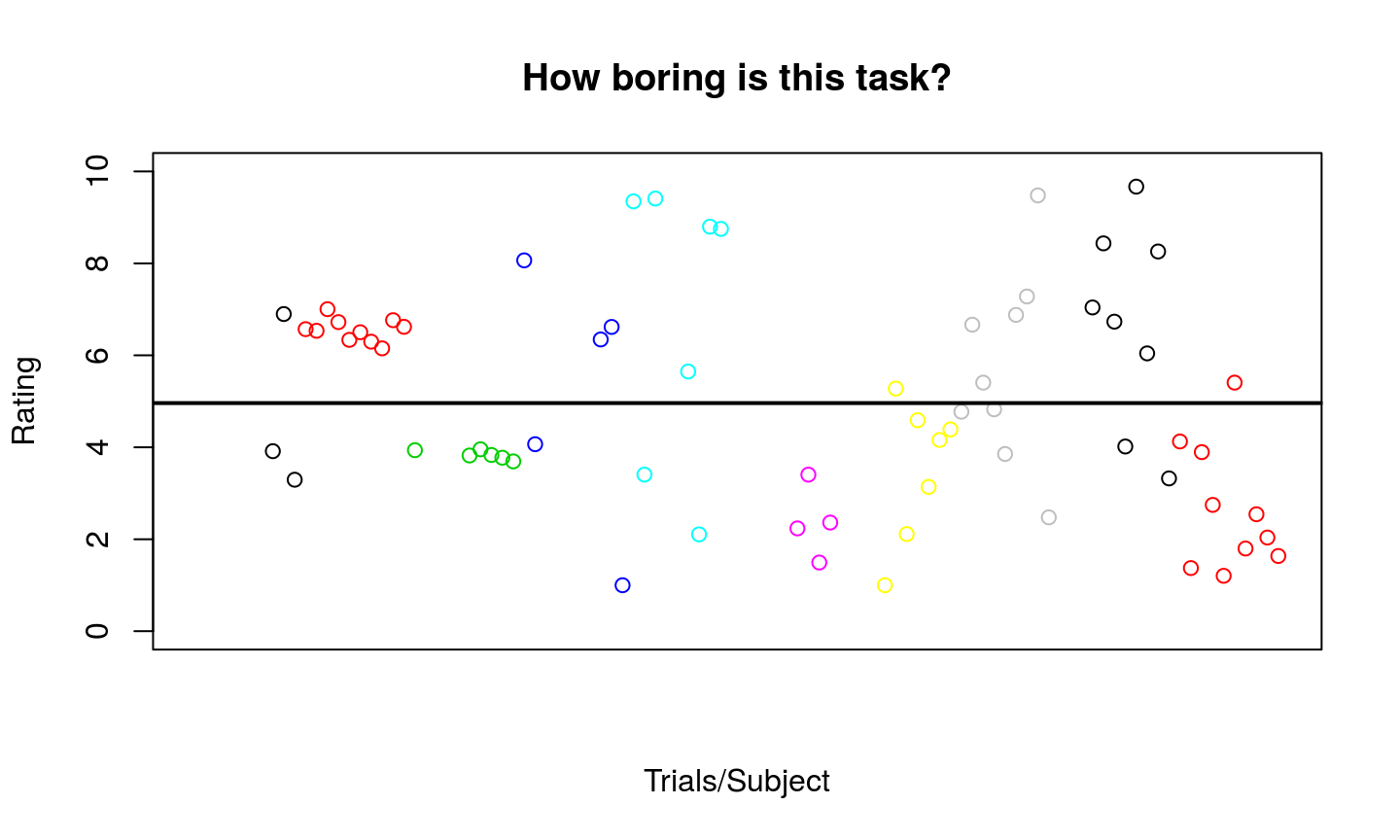## Unbalanced designs

Calculate mean of rating per subject, then model.

```summary.data2 <- aggregate(rating3,by=list(id=id),mean,na.rm=T)
summary.model2 <- lm(summary.data2\$x~1)     ```

Make a linear mixed model using subject as random effect

`mixed.model2 <- lmer(rating3~1+(1|id))   `

## Unbalanced designs

`display(summary.model2)`
```## lm(formula = summary.data2\$x ~ 1)
##             coef.est coef.se
## (Intercept) 4.81     0.52
## ---
## n = 10, k = 1
## residual sd = 1.65, R-Squared = 0.00```

## Unbalanced designs

`display(mixed.model2)`
```## lmer(formula = rating3 ~ 1 + (1 | id))
## coef.est  coef.se
##     4.85     0.53
##
## Error terms:
##  Groups   Name        Std.Dev.
##  id       (Intercept) 1.51
##  Residual             1.82
## ---
## number of obs: 69, groups: id, 10
## AIC = 299.8, DIC = 294.8
## deviance = 294.3```

## Unbalanced designs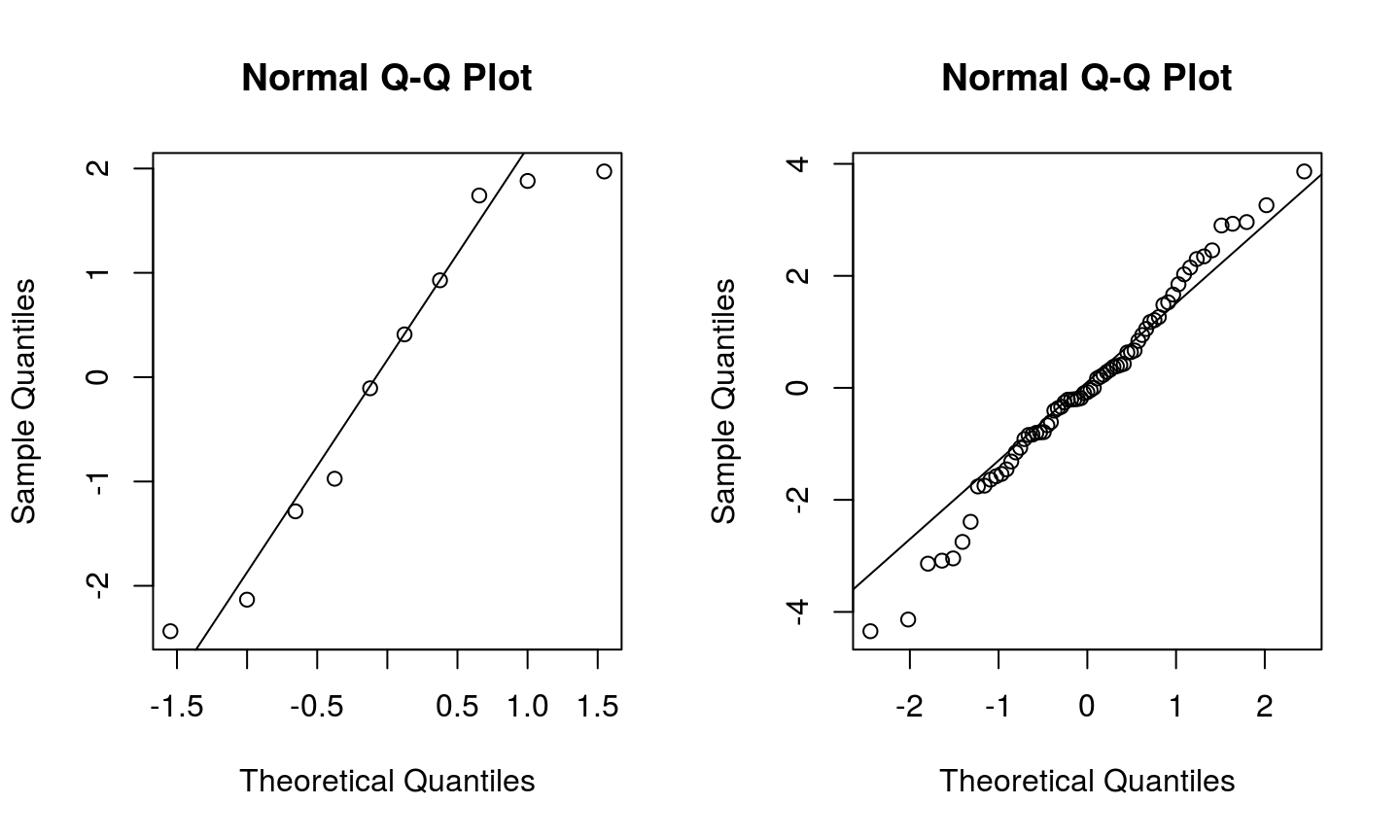## Unbalanced designs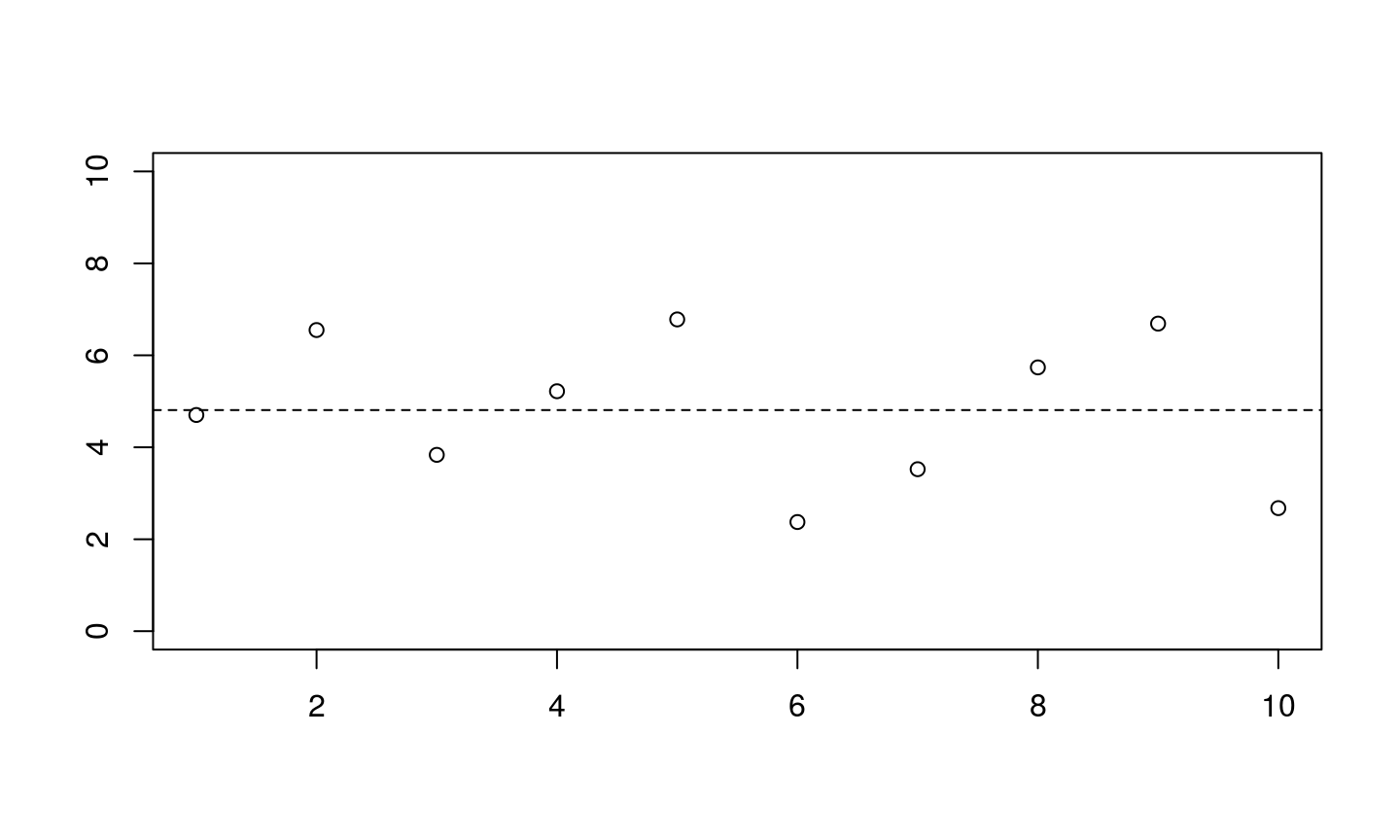## Unbalanced designs

`table(id)`
```## id
##  1  2  3  4  5  6  7  8  9 10
##  3 10  6  5  7  4  7  9  8 10```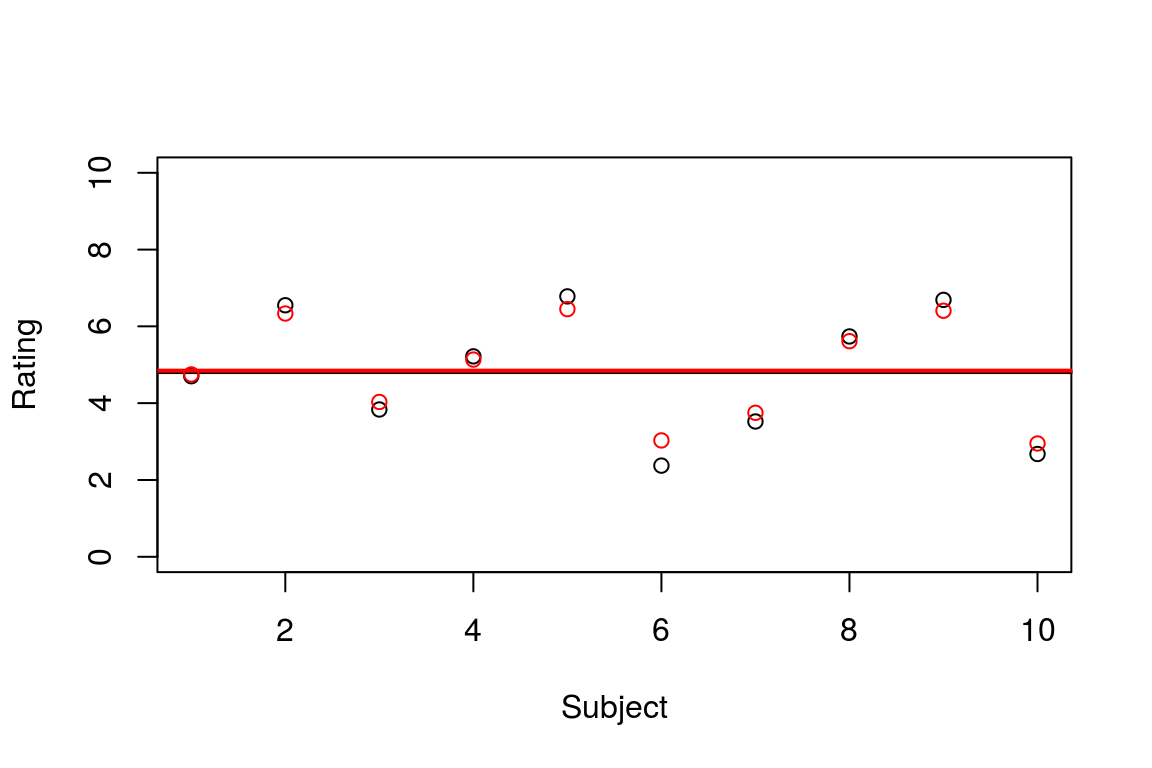## Estimation

### Very short summary

• Two-step estimation
• One-step estimation
• Pooled
• non-pooled
• partial pooled

## Note on terminology

• Fixed effects and random effects.
• Group level and individual level.
• 1st level and 2nd level.
• Within effects and between effects.
• Hieracical models, mixed-models, multilevel models, nested models, etc.

"Because of the conflicting definitions and advice, we avoid the terms "fixed" and "random" entirely, and focus on the description of the model itself […]"
- Andrew Gelman & Jennifer Hill

## Specifying models in R

Fixed effects are specified similar to lm/glm

`formula <- y ~ x  ## y as a function of x`
```y ~ 1           ## model the intercept for "y"
y ~ x           ## model the main effect of x and the intercept for y
y ~ x + 1       ## the same as above (+ 1 is implicit)
y ~ x + 0       ## model the main effect of x and no intercept
y ~ x - 1       ## the same as above
y ~ 0           ## doesn't model anything (Thanks Lau for including this!)
y ~ x + z       ## model the main effects x and z (and an intercept)
y ~ x:z         ## model interaction of x and z
y ~ x * z       ## model the main effects x and z and their interaction
y ~ x + z + x:z ## the same as above```

## Specifying random effects in lmer/glmer

```intercept.model           <- lmer(y ~ x + (1|id))
slope.and.intercept.model <- lmer(y ~ x + (x|id))
slope.model               <- lmer(y ~ x + (x-1|id))```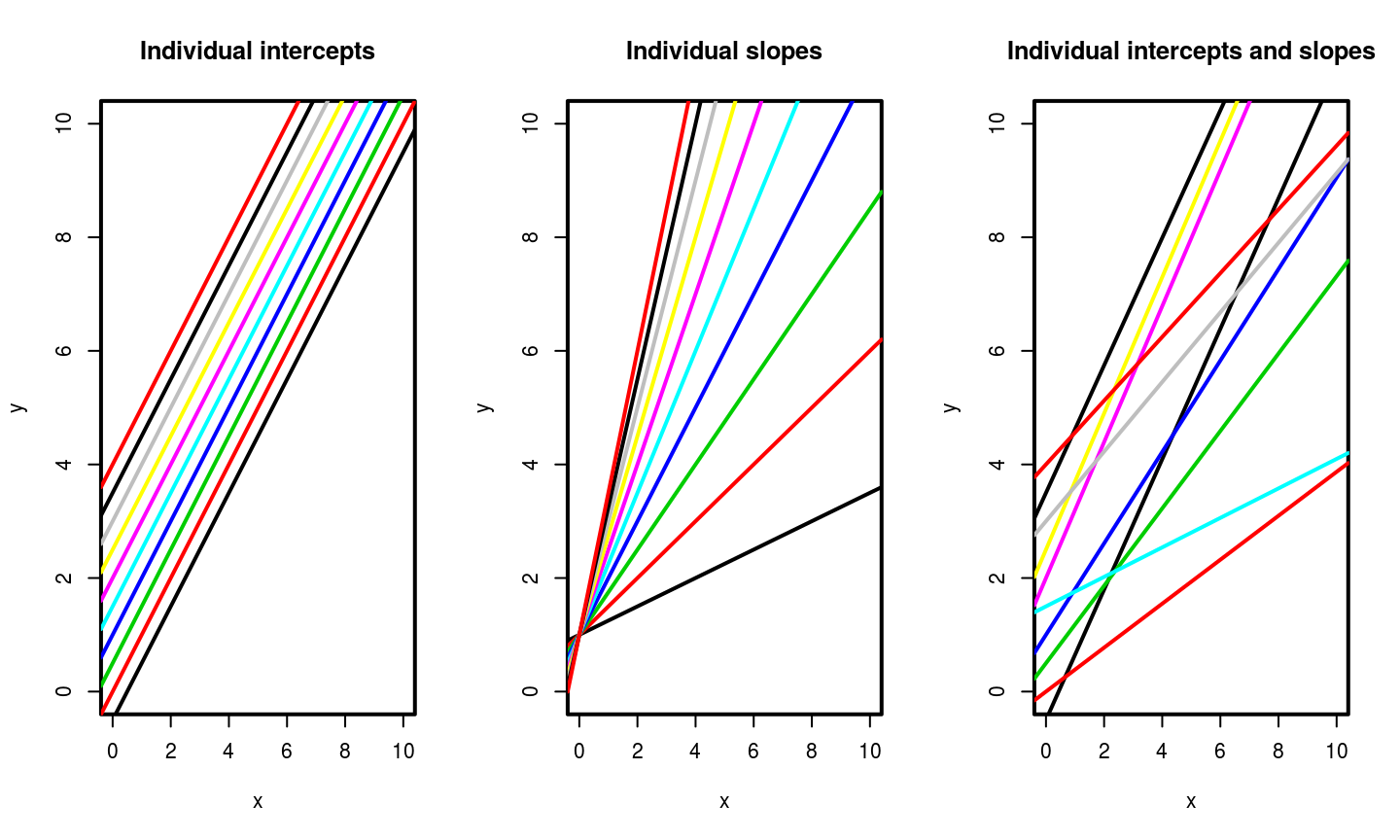## Example

`str(time.data)`
```## 'data.frame':    1200 obs. of  3 variables:
##  \$ rating: num  4.93 4.96 4.29 5.74 6.01 ...
##  \$ time  : int  1 2 3 4 5 6 7 8 9 10 ...
##  \$ id    : Factor w/ 20 levels "1","2","3","4",..: 1 1 1 1 1 1 1 1 1 1 ...```
`summary(time.data)`
```##      rating            time             id
##  Min.   : 1.094   Min.   : 1.00   1      : 60
##  1st Qu.: 3.599   1st Qu.:15.75   2      : 60
##  Median : 5.337   Median :30.50   3      : 60
##  Mean   : 5.236   Mean   :30.50   4      : 60
##  3rd Qu.: 6.771   3rd Qu.:45.25   5      : 60
##  Max.   :10.000   Max.   :60.00   6      : 60
##                                   (Other):840```